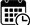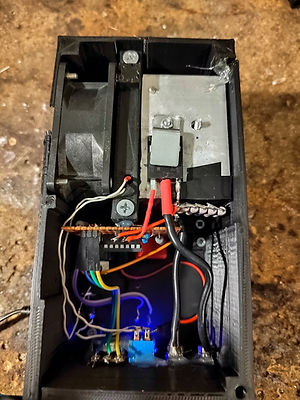top of page8th April 2023

Introduction

Recently I have built an adjustable dual rail power supply which I'll be using for my bachelor's thesis. What I need now is to test its efficiency for different amount of output current. I could have used some power resistors to load the power supply but that solution has many inconveniences; different current flow for different output voltages, temperature instability (resistor's resistance increases with temperature) and the need to calculate which resistors I need to load the power supply to certain load, etc...

Thankfully rather simple solution exists that uses a MOSFET as an adjustable load.img.1. - Constant current load basic schematics

The way this circuit works is really elegantly simple. Operational amplifiers (op-amps) are outputting such a signal to try to keep zero voltage difference between the input pins. In this schematics, every time when +input voltage is higher than -input, the output of the op-amp (U1) goes high. This opens up the transistor Q1 which then starts conducting current. As the op-amp voltage goes high, so does the current flowing and since we have a current flow across a resistor R1, there will be noticeable voltage drop on it. This voltage drop acts as a feedback for the op-amp. Once the current flow is large enough to create voltage on -input that matches the +input voltage, the op-amp is in the desired state and it will keep the output voltage at this level. The voltage on +input is simply adjusted with a potentiometer.

Let's see this on an example:

If we set the potentiometer to a state where the +input voltage is going to be 2V, the op-amp will open the gate of the transistor and it let current to rise so high to create the same 2V voltage drop on resistor R1. This happens when there are 2A of current flowing and at this current the circuit will be in a steady state no matter how much we change the supply input voltage.

You don't have to use 1Ohm resistor, but with 1Ohm the math is extremely simple. Since the current flow is simply equal to

I = Uinput+/1Ohm
A simple 1:1 voltage:current ratio.

You can use any different resistance and calculate the load current the same way I=Uinput+/R.

Build

The schematics on img.1. is slightly simplified, the real schematics I've followed while building the circuit is this:img.2. - Constant current final schematics

As you can see, there are a few modifications; a linear voltage regulator to output stable 5V which is then divided with 22k:10k ratio of R3 and RV1. This gives me maximum input+ voltage of around 1.6V. To set maximum load current to a bit higher value, I've used 6 parallel 1Ohms resistors for total of 166mOhm. 1.6V drop across such a resistance will occur at 9.6Amps. This is the maximum current the load can be adjusted to.

Resistor R5 is present to limit the output current of the op-amp not to exceed any ratings during the charging/discharging of the IRFP460' Gate.

Resistors R6,R4 and C3 are there just for stability although I suspect that they are not really needed.

After assembling the circuit on a perfboard I've placed the transistor on a heatsink with a fan powered by the 12V rail.img.3. - CC load view 1

img.4. - CC load view 2

img.5. - CC load view 3

For this I then printed a custom boximg.6. - CC load view 4

img.7. - CC load view 5

img.8. - CC load view 6img.9. - 3D model of the constant current load

As all my other 3D prints, this box was designed in Autodesk Fusion 360 software.

vid.1. - Constant current load test

From the video above you can see the load is fully adjustable from zero to maximum current (limited by my power supply to 3A). I have also varied the input load voltage so you can see the current flow is pretty much stable.

In conclusion I would say that having an adjustable current load is extremely handy and it was very simple to build a DIY version. The circuit is pretty much fool-proof, when powered from at least 12V, the circuit should work using any MOSFET, just make sure you're operating the MOSFET in the region in which it's safe. In datasheet you can see this region here:img.10. - IRFP460 safe operational areaimg.11. - IRFP3006 safe operational area

Unfortunately IRFP460 datasheet doesn't provide a DC safe region, only 10ms. It's clear that IRFP460 was not designed to operate in linear region. Thankfully IRFP460 has a big transistor dye which allows for lossy linear operation. On image #11 you can see an example transistor for which the datasheet provides a DC safe operation. As you can see there is not that big difference between 10ms and DC safe operation.

If you're planning to build the circuit and use lower voltage to power the op-amp, make sure you're using a logic level transistor like IRLZ44N, as the op-amp won't be able to output more than 5V (some op-amps are not "rail-to-rail", meaning that they won't even be able to provide the supplied voltage).

For now, the way this adjustable load works is perfect for me, although in the future I might add a microprocessor and load voltage feedback to also allow for constant power load and more precise adjustability.

bottom of page